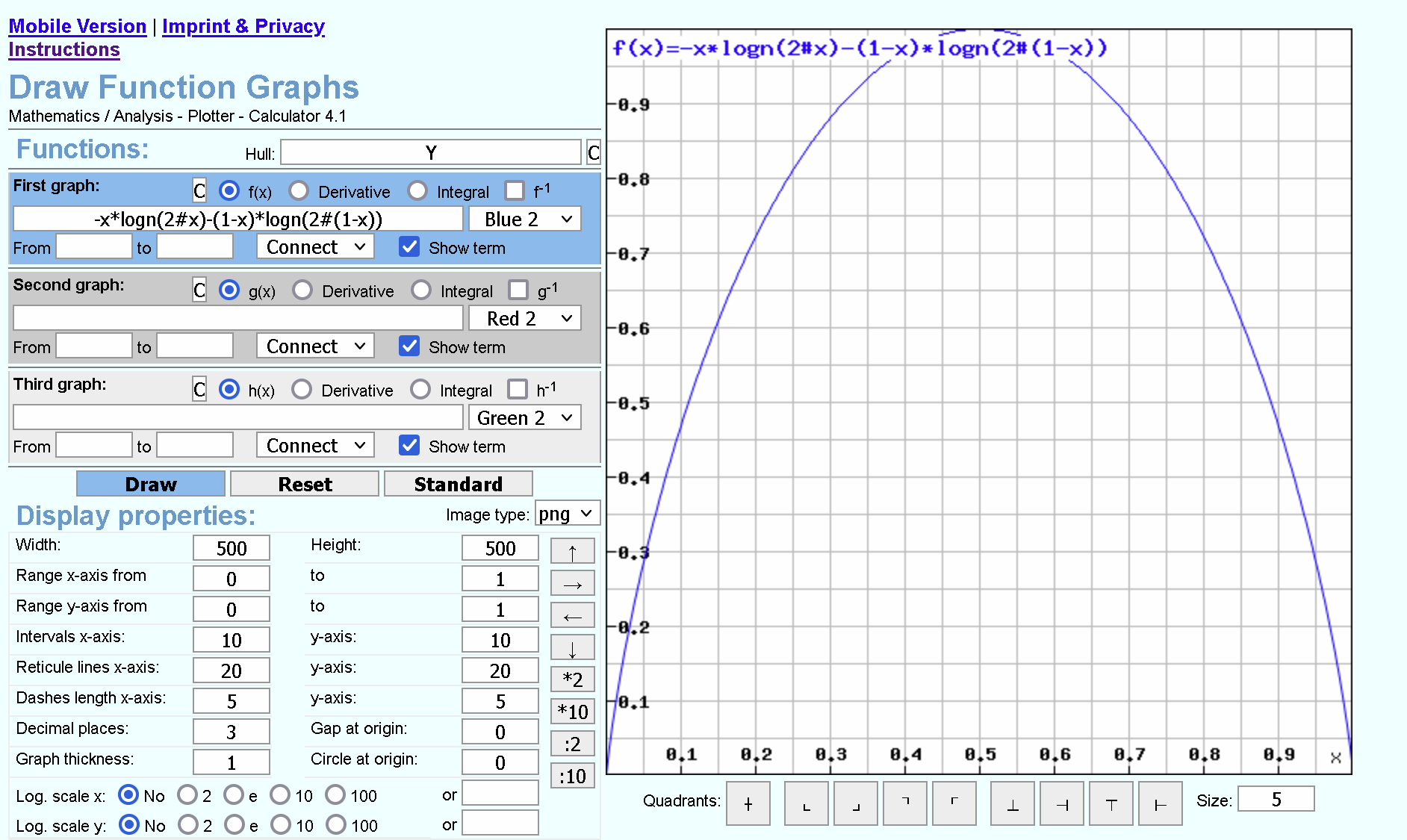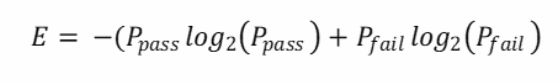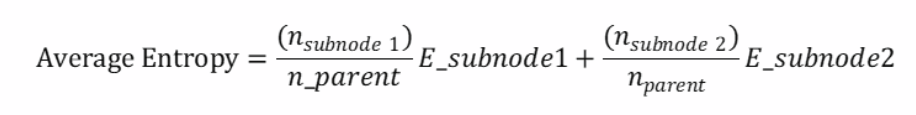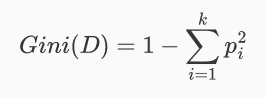Amount of information

there is in an event using the probability of the event. This is called “Shannon information,” “self-information,” or simply the “information,” and can be calculated for a discrete event x as follows:

• information(x) = -log2( p(x) )

Where log2() is the base-2 logarithm and p(x) is the probability of the event x.

The choice of the base-2 logarithm means that the units of the information measure is in bits (binary digits).

This can be directly interpreted in the information processing sense as the number of bits required to represent the event.

if the probability of finding something  is 1/2

then the number of bits needed is -log2(1/2)=-log2(1)+log2(2)=1

if the probability of finding something  is 1/4

then the number of bits needed is -log2(1/4)=-log2(1)+log2(4)=2

Entropy is the weighted average of information=-p*log2(p)-(1-p)log2(1-p)### There are two decisions to be made to form a decision tree

1) Information gain

a) Based on EntropyBased on Gini Impurity2) stop criteria (for stopping before reaching a pure leaf node)

a) reduction in impurity

b) minimum number of samples in node

c) Maximum depth

https://en.wikipedia.org/wiki/Machine_learning

### Decision Tree Calculations

https://medium.com/towards-data-science/decision-trees-explained-entropy-information-gain-gini-index-ccp-pruning-4d78070db36c

### Random Forest has two sources of randomness

1) random selection of predictors columns used to predict the predicted.

2) random selection of a subset of training data with replacement (Bootstrapping/Bagging),  Then evaluation using Out-Of-Bag subset.

For each bootstrap sample taken from the training data, there will be samples left behind that were not included. These samples are called Out-Of-Bag samples or OOB. The performance of each model on its left out samples when averaged can provide an estimated accuracy of the bagged models. This estimated performance is often called the OOB estimate of performance. These performance measures are reliable test error estimate and correlate well with cross validation estimates.

I like disabling the bootstrapping, which allows to find the best tree of features using the training data; then test that tree with the test data and finding the goodness of predictions in a classical confusion matrix.

Without bootstrapping, all of the data is used to fit the model, so there is not random variation between trees with respect to the selected examples at each stage. However, random forest has a second source of variation, which is the random subset of features to try at each split. “The sub-sample size is always the same as the original input sample size but the samples are drawn with replacement if bootstrap=True (default),” which implies that `bootstrap=False` draws a sample of size equal to the number of training examples without replacement, i.e. the same training set is always used.

setting `bootstrap=False` and using all the features does not produce identical random forests (may be because the random order of the top to bottom branches)

https://machinelearningmastery.com/bagging-and-random-forest-ensemble-algorithms-for-machine-learning/

https://stats.stackexchange.com/questions/534356/are-random-forests-trained-with-the-whole-dataset

https://en.wikipedia.org/wiki/Bootstrapping_(statistics)

https://stats.stackexchange.com/questions/354336/what-happens-when-bootstrapping-isnt-used-in-sklearn-randomforestclassifier

ٰData for an Introduction to Statistical Learning with Applications in R  https://cran.r-project.org/web/packages/ISLR/index.html

#### Videos

Topic Access

StatQuest: Decision Trees

Construct trees with GINI Impurity
for
Ratio interval
Ordinal
Nominal Level Data

No flaws detected

(17  min)

StatQuest: Decision Trees, Part 2 – Feature Selection and Missing Data

(5 min)

Decision Tree 1: how it works (9 min)

assumes that the tree end with pure sets !!!

Entropy discussed in a shallow way

Look at entropy from Thermodynamics point of view: https://www.grc.nasa.gov/www/k-12/airplane/entropy.html

https://en.wikipedia.org/wiki/Entropy_in_thermodynamics_and_information_theory

https://en.wikipedia.org/wiki/Entropy_(information_theory)

https://towardsdatascience.com/entropy-is-a-measure-of-uncertainty-e2c000301c2c

A Gentle Introduction to Information Entropy URL: https://machinelearningmastery.com/what-is-information-entropy/
Decision Tree (CART) – Machine Learning Fun and Easy (9 min) https://www.youtube.com/watch?v=DCZ3tsQIoGU

Decision Tree In R (46 min)

by Simplilearn

last 15 minutes not useful for students

Pruning Classification trees using cv.tree ( 15 min) https://www.youtube.com/watch?v=GOJN9SKl_OE
Machine Learning and Decision Trees ( first 15 minutes ) https://www.youtube.com/watch?v=RmajweUFKvM

#### Articles

Medium Topic Access
Tutorial Classification Trees https://daviddalpiaz.github.io/r4sl/trees.html
Tutorial A Complete Guide on Decision Tree Algorithm https://www.edureka.co/blog/decision-tree-algorithm/
Tutorial Decision Tree: How To Create A Perfect Decision Tree? https://www.edureka.co/blog/decision-trees/
Tutorial What is Overfitting In Machine Learning And How To Avoid It? https://www.edureka.co/blog/overfitting-in-machine-learning/
Tutorial Decision Tree in R with Example https://www.guru99.com/r-decision-trees.html
Tutorial Decision Tree and Random Forests https://www.analyticsvidhya.com/blog/2016/02/complete-tutorial-learn-data-science-scratch/
Tutorial Classification & Regression Trees http://www.di.fc.ul.pt/~jpn/r/tree/tree.html

Using “rpart”  library for CART

“classification and regression trees” (CART) relies on ‘recursive partitioning’ to identify patterns in the variance of response variables with respect to explanatory variables of interest. If the response is categorical, it creates classification trees.
If the response numeric, it creates regression trees.

We partition the response into the two most homogenous groups possible based on our explanatory variables (predictors). If categorical, this means splitting into two categories. In the case of a binomial predictor, we end up with two groups- each containing only a single category.

The initial split is found by maximizing homogeneity of variance within groups in the partition based on all all possible splits for each of the explanatory variables.

-=-=-=-=-=-=-=-=-=-=-=-=-=-=-=-=-

### `rattle::fancyRpartPlot() is good`

https://www.gormanalysis.com/blog/magic-behind-constructing-a-decision-tree/

``````library(rattle)
library(rpart.plot)
library(RColorBrewer)``````
``````library(rpart)
mytree <- rpart(
Fraud ~ RearEnd,
data = train,
method = "class"
)``````
``````# plot mytree
rattle::fancyRpartPlot(mytree, caption = NULL)``````

## -=-=-=-=-=-=-=-=-=-=-=-=-=-=-=-=-

### A package for all the  seasons Classification And REgression Training

51 models

http://topepo.github.io/caret/index.html

Since 11 April 2023: 261 total views,  1 views today